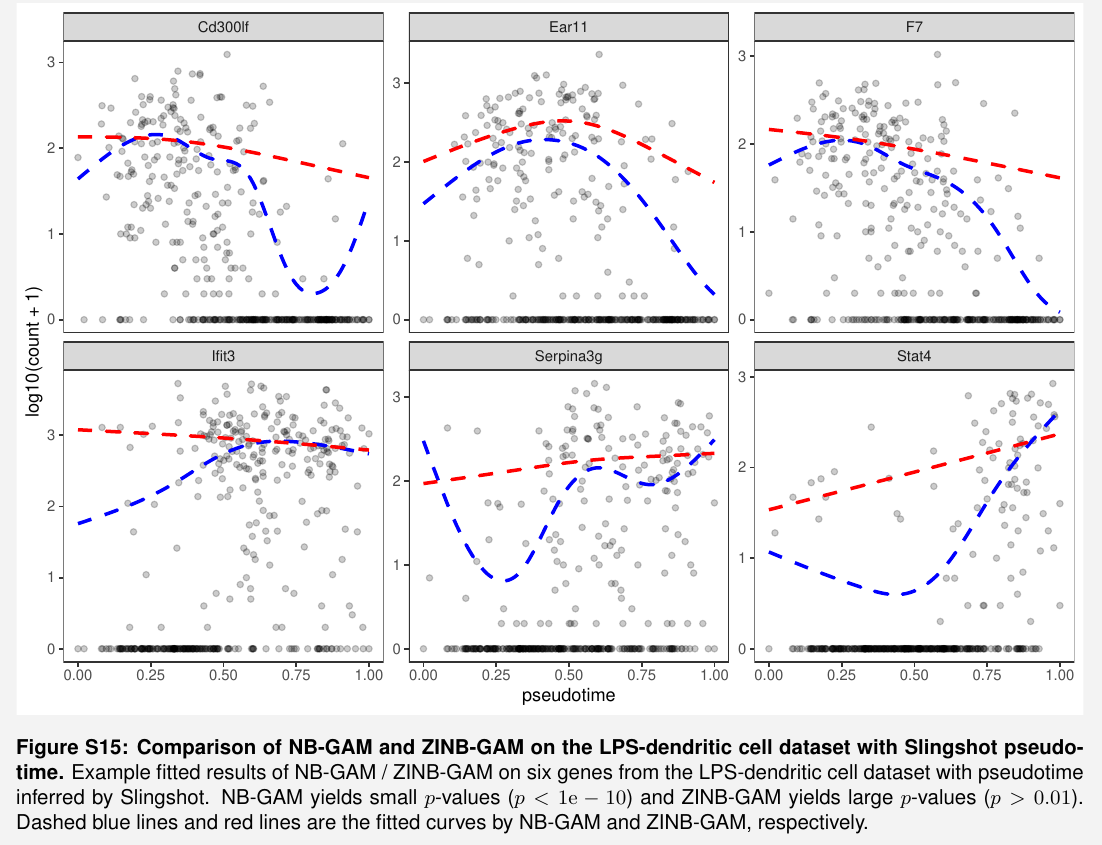# PseudotimeDE: Differential Gene Expression along Cell Pseudotime

##### Posted on Jul 27, 2023

If a gene’s mean expression changes along pseudotime, the gene is referred to as differentially expressed (DE) and is likely to play an important role in the underlying cellular process that gives rise to the pseudotime.

methods to identify DE genes: use the generalized additive model (GAM) to fit each gene’s expression level in a cell as a smooth-curve function of the cell’s inferred pseudotime.

• tradeSeq: use NB-GAM to model the relationship between each gene’s expression in a cell and the cell’s pseudotime.
• Monocle3-DE: use GLM.

other methods developed for identifying physical-time-varying DE genes from bulk RNA-seq time-course data.

## Methods

• $Y=(Y_{ij})$ an $n\times m$ gene expression count matrix, whose rows and columns correspond to $n$ cells and $m$ genes, respectively.
• $T = (T_1,\ldots, T_n)^T$: take $\bfY$ as input, a pseudotime inference method would return a pseudotime vector, where $T_i\in [0, 1]$ denotes the normalized inferred pseudotime of cell $i$. Note that $T_i$ is a random variable due to the random-sampling nature of the $n$ cells and the possible uncertainty introduced by the pseudotime inference method.

### Uncertainty estimation

subsample 80% cells in $\bfY$ for $B$ times to estimate the uncertainty of pseudotime $\bfT$

### PseudotimeDE model

use the NB-GAM model as the baseline model to describe the relationship between every gene’s expression in a cell and the cell’s pseudotime.

For gene $j$, its expression $Y_{ij}$ in cell $i$ and the pseudotime $T_i$ of cell $i$ are assumed to follow

$Y_{ij} \sim NB(\mu_{ij}, \phi_j) \log(\mu_{ij}) = \beta_{j0} + f_j(T_i)$

To account for excess zeros in scRNA-seq data that may not be explained by the NB-GAM, introduce a hidden variable $Z_{ij}$ to indicate the “dropout” event of gene $j$ in cell $i$, use the zero-inflated negative binomial-generalized additive model (ZINB-GAM)

$Z_{ij} \sim Ber(p_{ij}) Y_{ij}\mid Z_{ij} \sim Z_{ij}\cdot NB(\mu_{ij}, \phi_j) + (1-Z_{ij})\cdot 0\\ \log(\mu_{ij}) = \beta_{j0} + f_j(T_i)\\ \logit(p_{ij}) = \alpha_{j0} + \alpha_{j1}\log(\mu_{ij})$

### Statistical test and p-value calculation

To test if gene $j$ is DE along cell pseudotime, define the null and alternative hypotheses as

$H_0: f_j(\cdot) =0\quad\text{vs.} \quad H_1:f_j(\cdot)\neq 0$

### Pseudotime inference methods

apply two state-of-the-art methods, Slingshot and Monocle3-PI to inferring the cell pseudotime of each dataset

### DE analysis methods

compare PseudotimeDE with four existing methods for identifying DE genes along pseudotime/time-course from scRNA-seq data (tradeSeq and Mononcle3-DE) or bulk RNA-seq data (Impulse DE2 and NBAMSeq)

the fitting on real data looks not good enoughbut it seems just to show the difference between NB and ZINB

Published in categories Note